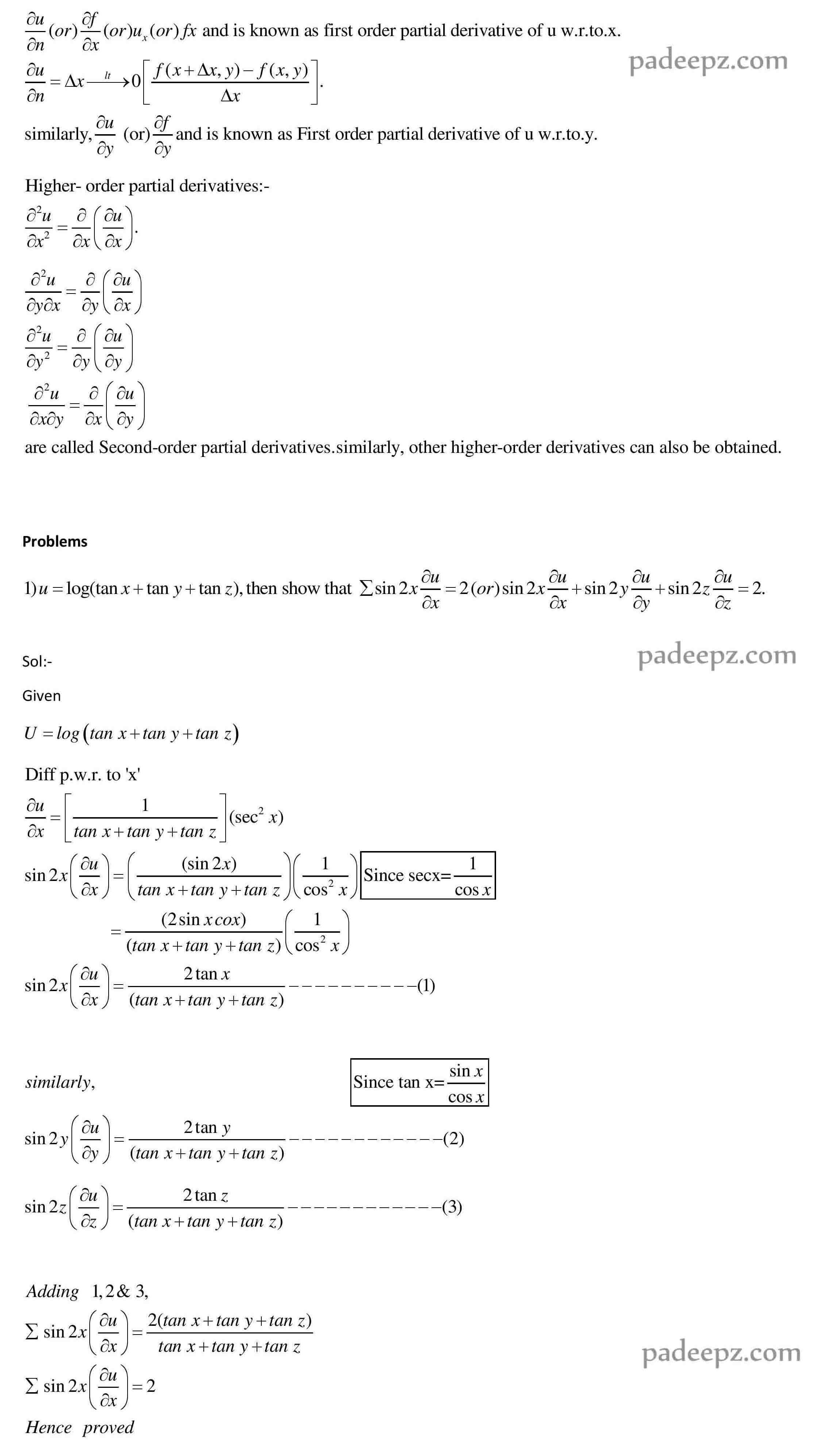# MA8151 Syllabus Engineering Mathematics 1

## OBJECTIVES : MA8151 Syllabus Engineering Mathematics 1

 The goal of this course is to achieve conceptual understanding and to retain the best traditions of traditional calculus. The syllabus is designed to provide the basic tools of calculus mainly for the purpose of modelling the engineering problems mathematically and obtaining solutions. This is a foundation course which mainly deals with topics such as single variable and multivariable calculus and plays an important role in the understanding of science, engineering, economics and computer science, among other disciplines.

### UNIT I DIFFERENTIAL CALCULUS MA8151 Syllabus Engineering Mathematics 1

Representation of functions – Limit of a function – Continuity – Derivatives – Differentiation rules – Maxima and Minima of functions of one variable.

#### UNIT II FUNCTIONS OF SEVERAL VARIABLES MA8151 Syllabus Engineering Mathematics 1

Partial differentiation – Homogeneous functions and Euler’s theorem – Total derivative – Change of variables – Jacobians – Partial differentiation of implicit functions – Taylor’s series for functions of two variables – Maxima and minima of functions of two variables – Lagrange’s method of undetermined multipliers.

##### UNIT III INTEGRAL CALCULUS MA8151 Syllabus Engineering Mathematics 1

Definite and Indefinite integrals – Substitution rule – Techniques of Integration – Integration by parts, Trigonometric integrals, Trigonometric substitutions, Integration of rational functions by partial fraction, Integration of irrational functions – Improper integrals.

###### UNIT IV MULTIPLE INTEGRALS MA8151 Syllabus Engineering Mathematics 1

Double integrals – Change of order of integration – Double integrals in polar coordinates – Area enclosed by plane curves – Triple integrals – Volume of solids – Change of variables in double and triple integrals.

###### UNIT V DIFFERENTIAL EQUATIONS MA8151 Syllabus Engineering Mathematics 1

Higher order linear differential equations with constant coefficients – Method of variation of parameters – Homogenous equation of Euler’s and Legendre’s type – System of simultaneous linear differential equations with constant coefficients – Method of undetermined coefficients.

### Padeepz E-Learning Materials MA8151 Engineering Mathematics 1

Padeepz E-Learning Materials MA8151 Engineering Mathematics 1 we have provided the sample materials in this page. If you like the sample and want to buy the full subject the procedure is also provided in this page.

#### Partial derivatives

partial derivative Introduction:

A partial derivative of a function of several variables is the ordinary derivative with respect to one of the variables, when all the remaining variables are kept constant. Consider a function u=f(x,y). Here , u is the dependent variable and x & y are independent variables. The partial derivative of u=f(x,y) with respect to x is the ordinary derivative of u w.r.to x, keeping y constant. It is denoted bySubject name Engineering Mathematics 1 Subject Code MA8151 Semester 1 Regulation 2017 Regulation

MA8151 Notes Engineering Mathematics 1

MA8151 Important Questions Engineering Mathematics 1

MA8151 Question Bank Engineering Mathematics 1

## One Reply to “MA8151 Syllabus Engineering Mathematics 1 Regulation 2017 Anna University”

1.Brunda says:

Upload maths refernce book MA8151 book